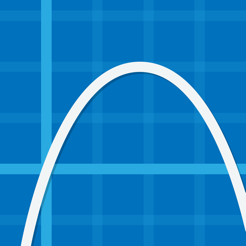## Screenshots

•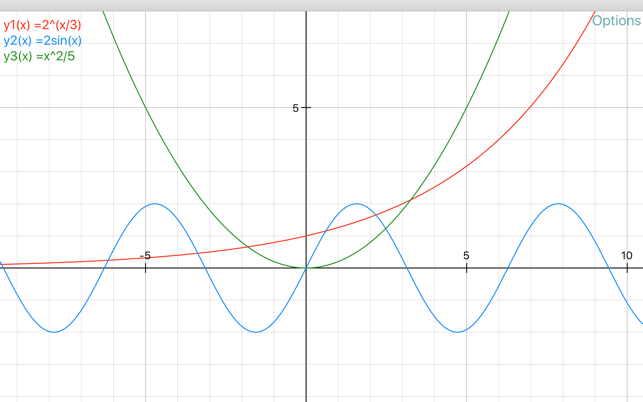••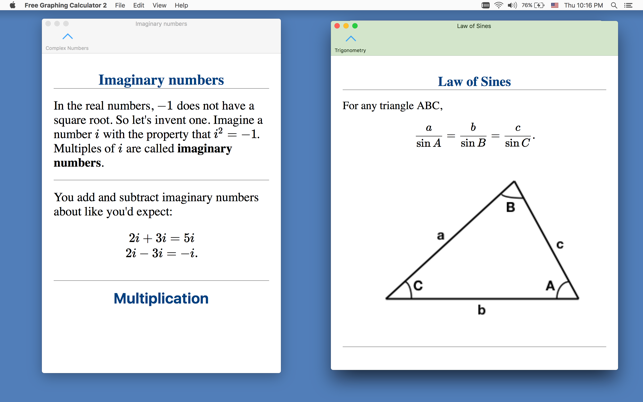•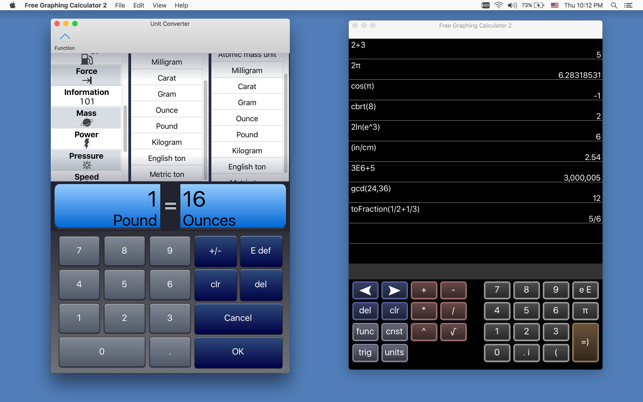•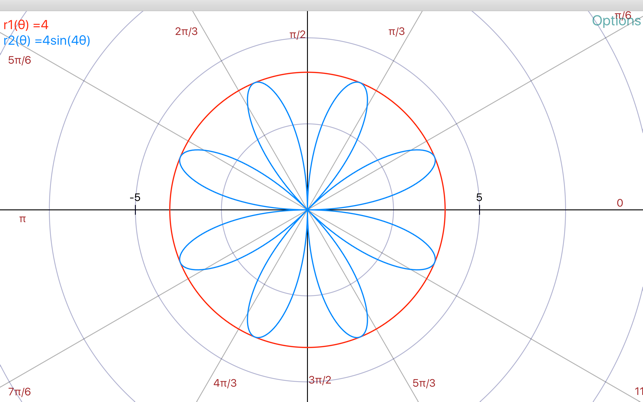## Description

The wildly successful iOS app, finally on Mac!

-- You can type most math, or use the on-screen keyboard.
-- Multi-windowing
-- Polynomial solver up to 10th degree
-- Wide range of settings for font sizes and graph line widths
-- Updates to this version will be more frequent, going forward
-- Matrix calculator more intuitive and is integrated with main calculator screen
-- Table has an "ask" mode where you enter input values and it gives you the outputs.

The Free Graphing Calculator app, re-written from the ground up:

-- Polynomial solver up to 10th degree
-- Wide range of settings for font sizes and graph line widths
-- Additional matrix/systems of equations material in reference, with more coming
-- Copy/Paste works in many places
-- Updates to this version will be more frequent, going forward

Does far more than most of the paid calculators out there . . . let alone the free ones.

Features:

1) Scientific Calculator. Simple to grasp and easy to use, but powerful features are available when you need them. Available functions include the following:

• the usual arithmetic functions and exponentiation.
• square root, cube root, nth root, natural log, log base 10, log of arbitrary base, absolute value, factorial, permutations (nPr), combinations (nCr), modulus, random integer, bell curve, cumulative normal distribution, decimal to fraction.

2) Graphing. Capabilities:

• Graph up to four equations at once.
• Graphs are labeled.
• You can drag the graph or pinch to zoom in or out.
• Calculator can find roots and intersections.
• Graph in polar coordinates.
• Graph parametric equations
• Can graph implicit functions, such as x^2+y^2-4=0. Most calculator apps can't do this!

3) A unit converter. With a tap, you can enter the result of your conversion into the calculator. Currently converts different units of the following: acceleration, angle, area, density, distance, energy, force, mass, power, pressure, speed, temperature, time, and volume. Great for doing physics homework!

4) Constants for scientific calculations -- speed of light, strength of gravity at Earth's surface, etc. etc. etc. Tapping on a constant will insert it into your calculation -- i.e, you don't have to key in the value. Again, great for doing physics homework!

5) It can make a table of the values of any function you care to enter. You can choose the starting x value of the table, as well as how much x increases for each successive row.

6) Help screens linked directly to many of the available functions and constants. Tap the disclosure arrow to see the definition.

7) Forgot the quadratic formula? Or the double-angle formulas for sine and cosine? The math/science reference hits the high points of various subjects. Currently includes algebra, differential and integral calculus, geometry, trigonometry, vectors, vector calculus, and classical mechanics.

8) Keep track of significant figures [AKA sig figs]

9) Statistics -- enter data and make a histogram, box and whisker plot, or scatter plot with optional regression line.

If you are viewing this in iTunes, you will see five iPhone screenshots and five iPad screenshots. But even ten shots don't come close to showing everything this calculator can do.

I'd love to hear your comments or suggestions. You can write me at mathscixyzgraphcalc@gmail.com -- but without the xyz. Thanks!

Version 10.3

Math layout
Derivatives

## Ratings and Reviews

c1nnam0nBASS ,

### Won’t Open.

The application won’t open. Everytime I click this app a page shows up,” Application quit”.

## Information

Seller
William Jockusch
Size
16.4 MB
Category
Education
Compatibility

OS X 10.11 or later, 64-bit processor

Languages

English

Age Rating
4+
Price
Free
In-App Purchases
•